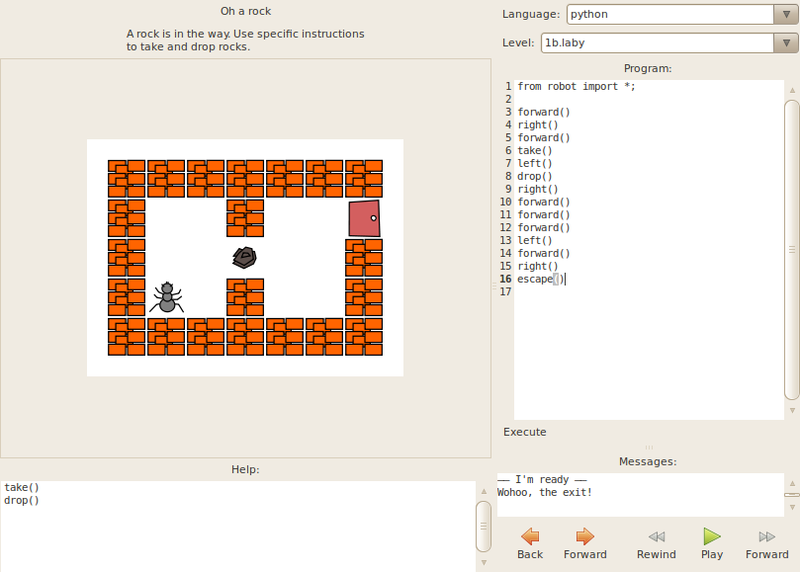# Laby Program

## Introduction to Laby program:

Laby is a small program to learn how to program with ants and spider webs. We have to move the ant robot out of a labyrinth and avoid spider webs. First level of Loby is tricky but easy. Help of while loops, of function definitions. It also needs acquire good skills at if conditionals. The programming language is set to Python in DoudouLinux and programming instructions names can be translated so that child can understand.

## Feature of the Laby Program:

• It helps to learn programming wit ants and webs.
• Programming instruction a names can be translated to child language so that they easily understand.
• Programming with Laby is simple
• While using Laby any one can learn python, C, OCaml and Java.

### Laby Programming Code:

```{`
(* ocaml wall hugger for laby *)
let ant =
(* go straight ahead til we hit something *)
while (Robot.look () = Void) do
Robot.forward ();
done;
(* main wall hugin' loop *)
while not (Robot.look () = Exit) do
if (Robot.look () == Void)
then (
Robot.forward ();
Robot.right ();
);
if (Robot.look () == Rock)
then (
Robot.take ();
Robot.forward ();
Robot.left ();
Robot.left ();
Robot.drop ();
Robot.left ();
);
if (Robot.look () == Wall) or (Robot.look () == Web)
then
Robot.left ();
done;
Robot.door_open ();
;;
`}```

## Laby Program